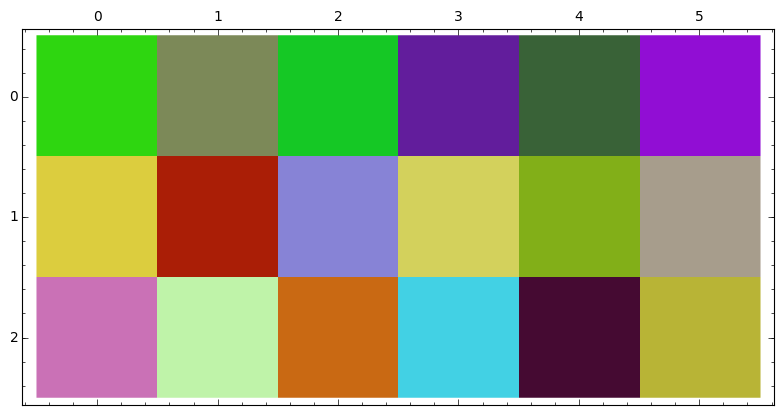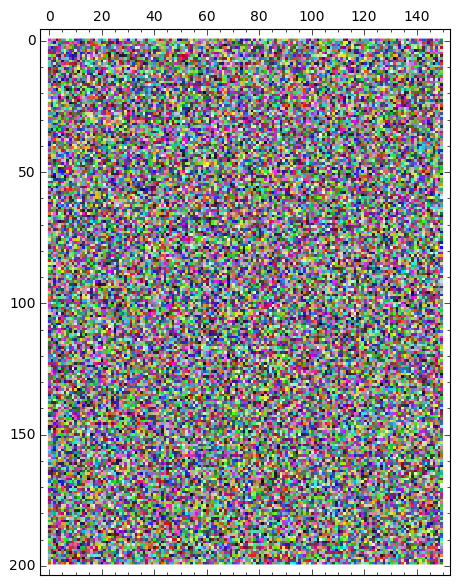# nxm plot from nxm color matrix 'no interpolating'

I want to create an nxm png from a given nxm matrix of html_Colors with 'no interpolating' because the adjacent points have dissimilar colors, If possible with enlargable square pixels. It feels like it should be trivial, but I have tried a long time and remain clueless. Thanks! --Herb

edit retag close merge delete

Sort by » oldest newest most votedI remain clueless about what you are asking, could you please be more clear? When you say $m\times n$ png, do you mean the size in pixels? What exactly do you mean with "enlargable square pixels"?

However here is an answer to a possible interpretation that was interesting to think about. hth

If your input is a matrix of colors in html format, eg, something that may have been the output of rand_color_matrix (see below).

def rand_html_color():
return '#'+''.join([hex(randint(0,255))[2:].zfill(2) for i in range(3)])
def rand_color_matrix(m,n):
return [[rand_html_color() for j in range(n)] for i in range(m)]


Then you can use sage's matrix_plot to generate a png by using plot_color_matrix defined as:

def plot_color_matrix(M):
L=sorted(flatten(M))
nrows=len(M)
ncols=len(M)
m = matrix(ZZ, nrows, ncols)
for (i,row) in enumerate(M):
for (j,color) in enumerate(row):
m[i,j] = L.index(color)
return matrix_plot(m,cmap=L)


Sage's matrix_plot generates an $m\times n$ array of colored squares where the color of square $i,j$ corresponds to the entry $i,j$ of a given matrix. If you provide a list of colors, say L, then color L[k] is used for entry $i,j$ if the "rank" of that entry is k. The idea behind plot_color_matrix is to get the matrix of colors, generate a list of sorted colors and then just construct a matrix that will make matrix_plot color the entries exactly as they were given in M.

Sample session and output:

sage: %attach color_matrix.sage
sage: m=rand_color_matrix(3,6)
sage: m
[['#2ed610', '#7c8958', '#15c825', '#621d9c', '#396237', '#910ed4'],
['#dccd3e', '#aa1e06', '#8783d6', '#d3d15c', '#82af18', '#a79d8c'],
['#ca71b6', '#bff3a9', '#c96913', '#42d1e4', '#450a32', '#b8b436']]
sage: plot_color_matrix(m)A larger example:

sage: plot_color_matrix(rand_color_matrix(200,150))more